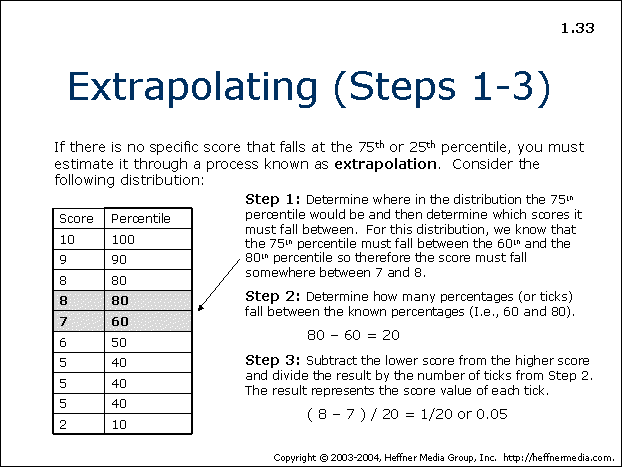# 33: Extrapolating: Distribution, Percentages## Extrapolating (Steps 1-3)

If there is no specific score that falls at the 75th or 25th percentile, you must estimate it through a process known as extrapolation. Consider the following distribution:

Step 1: Determine where in the distribution the 75th percentile would be and then determine which scores it must fall between. For this distribution, we know that the 75th percentile must fall between the 60th and the 80th percentile so therefore the score must fall somewhere between 7 and 8.

Step 2: Determine how many percentages (or ticks) fall between the known percentages (I.e., 60 and 80).

80 – 60 = 20

Step 3: Subtract the lower score from the higher score and divide the result by the number of ticks from Step 2. The result represents the score value of each tick.

( 8 – 7 ) / 20 = 1/20 or 0.05